# Understanding the "Futures Trading"

## 10.1 - The Pricing Formula

You would likely be introduced to futures pricing formulas if you took a traditional course on Futures trading. We have chosen to discuss it later, so we will not be introducing it at the beginning of our course on Futures trading. It is easy to see why. If you trade futures using technical analysis (which I assume most of you do), then you don't need to know how futures are priced. However, a working knowledge would be helpful. If you want to trade futures using quantitative strategies like Index Arbitrage or Calendar Spreads, then you will need to be able to do so. We will actually have a module on 'Trading Strategies' in which we will discuss some of these strategies. This chapter will also serve as a foundation for the future modules.

You may recall that in the previous chapters, we often discussed the 'Futures Pricing Formula as the main reason for the difference between spot and futures prices. It is now that I think it is time to remove the veil and reveal the 'Future Pricing Formula.

We know that the value of a futures instrument is derived from its underlying. We also know that futures instruments move in tandem with their underlying. The futures price will fall if the underlying price drops, and vice versa. The underlying price and futures prices are different and not the same. As I write this, the Nifty Spot is trading at 8,845.5, while the current month contract is trading below at 8,854.7. Please refer to the snapshot below. The difference between the spot and futures prices is "basis". The spread for the Nifty example is 9.2 points (8854.7 to 8845.5).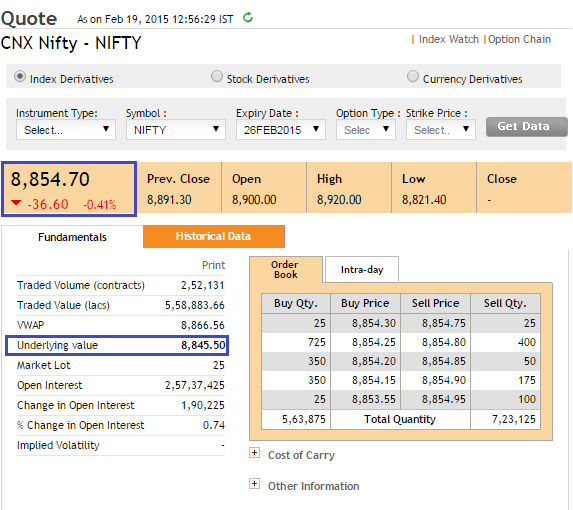The Spot-Future Parity' is responsible for the price difference. Spot future parity is the difference in spot and futures prices that results from variables like interest rates, dividends or expiry time. It is simply a mathematical expression that equates the futures price with its underlying price. This formula is also called the futures price formula.

The futures pricing formula is simple:

Futures price = Spot Price *(1+r f)-d

Where?

r F = Risk-free Rate

d - Dividend

You should note that 'r f' refers to the risk-free rate you can earn for the whole year (365 day). However, considering the expiry at 1, 2, and 3, one might want to scale it proportionally for other time periods than the exact 365 day period. A more general formula is -

Futures price = Spot Price * [1+ f* (x/365)] - d

Where?

x = Number of days until expiry.

The 91-day Treasury bill of the RBI can be used as a proxy to the short term risk-free interest rate. The same information can be found on the RBI's homepage, as shown below in the screenshot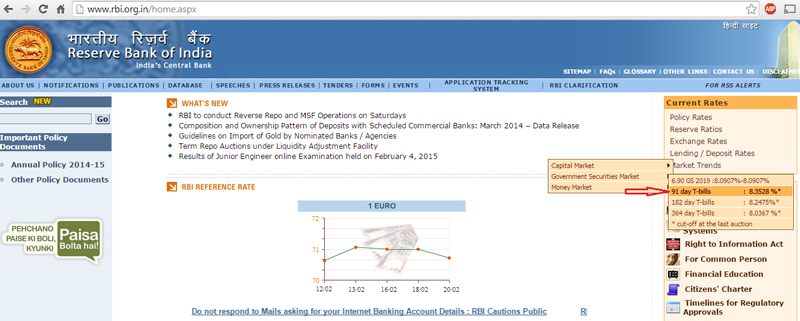The current rate is 8.3528%, as you can see in the above image. Let's look at a pricing example to keep this in mind. Let's say Infosys spot trades at 2,280.5 and has 7 days left to expire. What price should Infosys' current month futures contract look like?

Futures price = 2280.5 * (1+8.3528.% (7/365)]-0

Note that Infosys does not expect to pay any dividend in the next 7 days. Therefore, I have assumed a dividend of 0. The future price is 2283 if we solve the above equation. This is the fair value of futures. The actual futures price, as you can see in the image below, is 2284. The "Market Price" is the actual price at which futures contracts trade.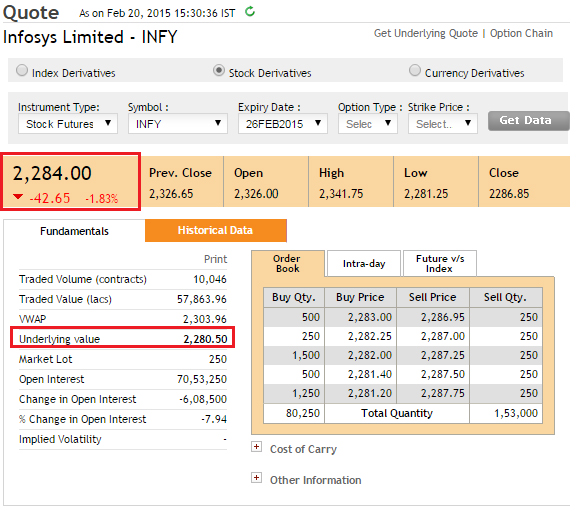Market costs like transaction fees, taxes, margins, etc. are the main reason for the difference in fair value and market prices. The fair value is a measure of where futures should trade at a given risk-free rate and expiry date. Let's look at the futures prices for far and mid-month contracts.

Mid-month calculation

Number of days until expiry = 34 (as contract expires on 26 March 2015).

Futures Price = 2280.5 multiplied by (1+8.3528.% ( 34/365),) - 0

= 2299

Far-month calculation

Number of days until expiry = 80 (as contract expires on the 30 th April 2015.

Futures Price = 2280.5 multiplied by (1+ 8.3528.%( 80/365),) - 0

= 2322

Let's take a look at actual market prices from the NSE website -

Snapshot Infosys's mid-month contract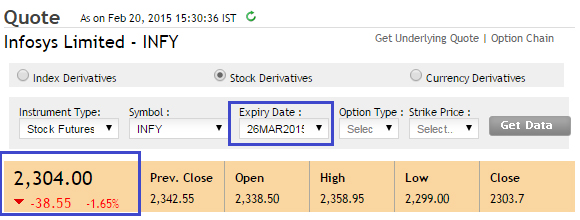Snapshot Infosys's mid-month contract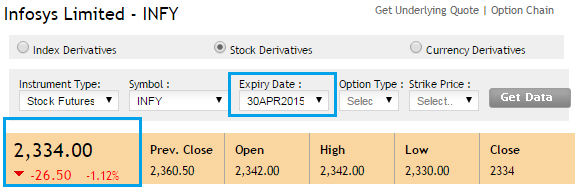There is clearly a difference in the market price and the calculated fair value. This could be attributed to the costs. The market may also be taking into account financial yearend dividends. The key thing to remember is that the gap between fair value and market value increases as the days until expiry.

This leads to , another commonly used market term. is short for discount or premium.

If futures are trading higher than spot, which mathematically speaking corresponds to the natural order, then the futures market will be called premium. Although 'Premium" is used in Equity derivatives markets the commodity derivatives market prefers to refer to the same phenomenon under the name'Contango'. Both premium and contango refer to the same thing - Futures trade higher than the Spot.

Below is a plot showing the Nifty spot and its futures for January 2015. As you can see, the Nifty futures traded above the spot throughout the entire series.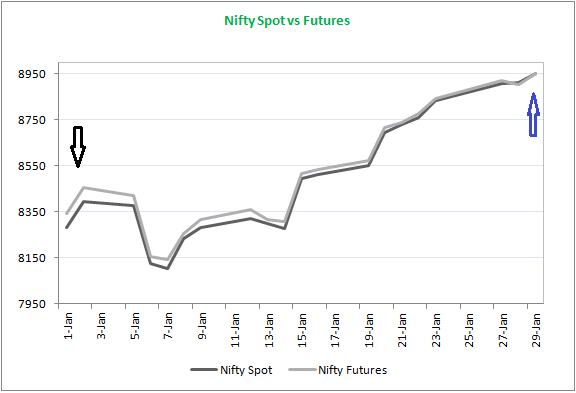These are the points that I want to emphasize.

1. The spread between spot and futures at the beginning of the series is high (highlighted with a black arrow). This is due to the high number of days until expiry, which is why the x/365 factor used in futures pricing formula is so high.
2. Throughout the series, the futures were at the top of the list.
3. The futures and the spot have converged at the end of the series, highlighted by a blue Arrow. This happens every time. No matter if the future is at a premium, or at a discount on expiry day, the futures will always converge.
4. If you hold a futures contract and fail to close the position before expiry, the exchange will automatically cancel the position. The spot price will be determined as the spot price of futures and spot on the day of expiry.

The spot may trade more than the futures, but not always. Sometimes, the spot may trade at a discount to the futures. This is mainly due to short-term demand and supply imbalances. When futures are trading at a discount to spot, this is called the "backwardation". The "backwardation" is the term used to describe the situation in the commodities industry.

## 10.2 - Practical Application

Let's put the futures price formula to good use before we close this chapter. As I mentioned, the futures pricing formula is very useful if you want to trade using quantitative trading techniques. The following discussion is a glimpse into trading strategies. All of these topics and more will be discussed in detail when we begin the module "Trading Strategies". Take a look at this:

Wipro Spot = 653

R f8.35%

x = 30

d = 0.

This means that futures should trade at -

Futures Price = 653* (1+8.35% (30/365)) + 0

= 658

To account for market charges, futures should trade in the range of 658 to 658. What if the futures contract trades at a dramatically different price? Let's take 700. This is clearly a trade. The spread between spot and futures should be 5 points. However, market imbalances have caused it to rise to 47 points. This spread can be captured by placing a trade.

This is how you can do it: Since the future contract trades above its fair price, we refer to the futures market prices as high relative to its fair worth. Or, you could say that the spot trades cheaper relative to futures.

In any type of spread trade, the thumb rule is to purchase the less expensive asset and sell it. This means that we can simultaneously sell Wipro Futures and buy Wipro on the spot market. Let's plug in the numbers to see how it goes.

Buy Wipro in Spot @ 653

Sell Wipro in Futures @ 700

We now know that the spot and futures both converge on the expiry date (refer the Nifty graph above). Let's assume that the futures and spot converge at a random value of 675, 645 and 715 to identify the trade.

Expiry ValueSpot Trade P&L (Long).Futures Trade P&L (Short).Net P&L
675675 - 653 = +22700-675 = +25+22 + 25 = +47
645645 - 653 = +08700-645 = +55-08 +55 = +47
715715 - 653 = +62700 - 715 = 15+62 - 15 =+47

You can see that once you have executed the trade at your expected price, you have effectively locked in the spread. Profits are guaranteed, regardless of market direction at expiry. It is a good idea to make a deal with your futures broker just before expiry. This would mean that you sell Wipro in spot and then buy Wipro back in Futures.

This type of trade between futures and spot to extract and profit is also known as the Cash and Carry Arbitrage.

## 10.3 Calendar Spreads

The cash & carry arbitrage is simply extended to the calendar spread. A calendar spread is a way to profit from the spread between futures contracts with the same underlying but different expiries. This is what we will do if we continue to use the Wipro example.

Wipro Spot trades at 653

Fair value of current month futures (30 days before expiry) = 658

Actual market value for current month futures = 700

Fair value of mid-month futures (65 days before expiry) = 663

Actual market value for mid-month futures = 665

The above illustration clearly shows that the current month futures contract trades at a premium to its theoretical fair value. The mid-month contract trades close to its fair value estimate. These observations lead me to assume that the current-month contract's basis will eventually shrink and that the mid-month contract will trade at its fair value.

The current month contract seems to be more expensive than the mid-month contract. We sell the more expensive contract to buy the cheaper one. The trade setup is to buy the mid-month futures contract @ \$665 and then sell it @ 700.

How do you see the spread? The spread is the difference in future contracts, i.e 700 vs 665 = 35 points.

This is how the trade was set up to capture spread.

Buy the current month futures @ 700

Purchase the mid-month futures at 665

Note that you will be selling and buying the same underlying futures with different expiries. Margins are significantly reduced because this is a hedged position.

After initiating the trade one must wait for the expiry of the current month's futures. The spot and current month's futures will be converged to one price after expiry. It is logical to unwind the trade before expiry.

Let's take a random set of scenarios and see how it turns out.

Expiry ValueP&L for the current month (Short).P&L Mid-Month (Long).Net P&L
660700 - 666 = +40660-665 = -5+40 - +5 = +35
690700-690 = +10690-665 = +25+10 +25 = +35
725700-725 = -25725-665 = +60-25 + 60 = +35

Remember, however, that the key assumption we made is that i.e. The mid-month contract will be close to its fair price. This is what I have seen in trading.

Please remember that the chapter on spreads is only a glimpse into the world trading strategies. These strategies will be discussed in a separate module that will provide an in-depth analysis of how to professionally implement them.

## To Summarize

1. Futures pricing formula says that the Futures price = spot price *(1+R f (x/365).)
2. The spread, or the basis, is the difference between spot and futures.
3. The "Theoretical Fair Value" is the futures price, as calculated by the pricing formula.
4. The'market price' is the price at which futures trade on the market.
5. The theoretical fair value for futures and the market value should generally be approximately equal. There could be some variance due to associated costs.
6. The futures are said to be in premium if it is easy to spot. Otherwise, they are said to be discounted
7. In commodity parlance, Premium = Contango, and Discount = Backwardation
8. Cash and Carry is a spread that allows one to buy on the spot and then sell in the futures.
9. Calendar spread is an extension to a cash and carry in which one purchases a contract and simultaneously buys another contract (with a differing expiry but the same underlying).